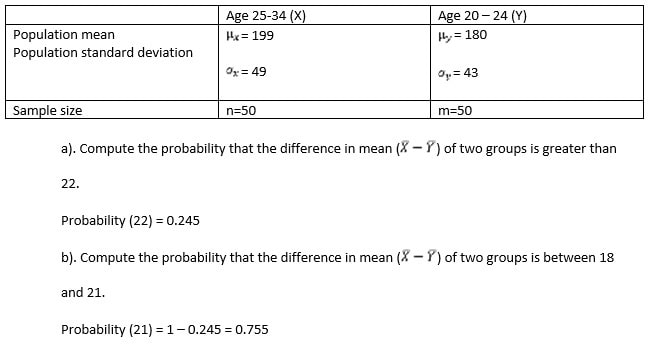# Biostatistics Assignment Question

Biostatistics questions

1. Data collected by the NCHS shows the percentage of adults told they have hypertension is 23.53 percent. If we select a simple random sample of 20 US adults and assume the probability that each has been told they have hypertension is .24.

This survey also shows that 32 percent indicated that they have been tested for HIV. Consider a simple random sample of 15 adults selected at that time. Find the probability that the number of adults who have been tested for HIV in the sample would be:

1. Three = 0.234
2. Less than 5 = 0.892
3. Between 5 and 9, inclusive =0.172
4. More than 5 but less than 10 = 0.871
5. 6 or more = 0.292

2. An investigator looked at the occurrence of RCH, a benign retinal tumor, in people with von Hippel-Lindau disease.(VHL) The researchers found the number of RCH tumor incidents followed a Poisson distribution with λ = 4 tumors per eye with patients with VHL. Using this model, find the probability that in a randomly selected patient with VHL:

1. There are exactly 5 occurrences of tumors per eye = 0.162
2. There are more than 5 occurrences of tumors per eye = 0.172
3. There are fewer than 5 occurrences of tumors per eye = 0.428
4. There are between 5 and 7 occurrences of tumors per eye, inclusive = 0.827

3. Calculate the following both

• Manually AND
• Using SPSS ……show SPSS printout

For a subject (29 year old male) in a study, acetone levels were normally distributed with a mean of 870 and a standard deviation of 211 ppb. Find the probability that on a given day the subjects acetone level is:

1. Between 600 and 1000 ppb = 0.104
2. OVER 900 ppb = 0.545
3. Under 500 ppb = 0.367
4. Between 900 and 1100 ppb = 0.156
5. Over 900ppb = 0.111

SPSS calculation,

4. Consider the following on serum Cholesterol levels of males.